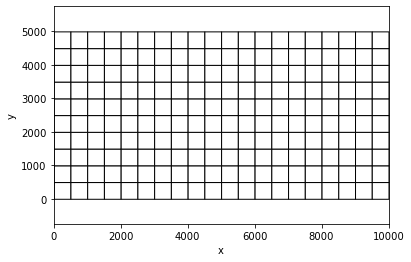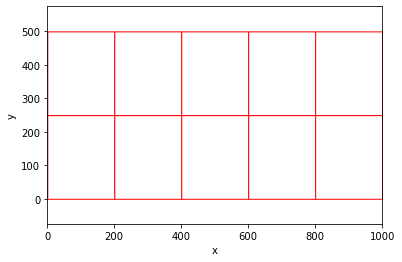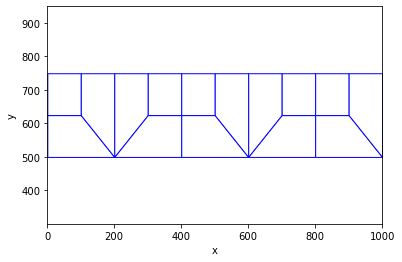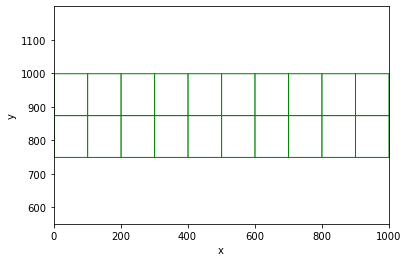# Basic Meshing API

## The most basic mesh to run with Salvus

This exercise shows the most basic mesh with all necessary commands to put material properties and boundaries ready to run with Salvus.

Copy
# initialize notebook
%matplotlib inline
import matplotlib.pyplot as plt
import numpy as np

plt.rcParams["figure.figsize"] = (10, 8)

### 2D

Set basic input parameters for a rectangular domain with homogeneous material properties.

vp = 1700  # p-wave velocity in m/s
vs = 1000  # s-wave velocity in m/s
rho = 2700  # density in kg / m^3

max_x = 10000  # domain size in x direction
max_y = 5000  # domain size in y direction

fmax = 1.0  # maximum frequency in Hz
elements_per_wavelength = 2.0  # resolution criterion

Compute element size and number of elements needed according to the resolution criterion.

hmax = vs / fmax / elements_per_wavelength
nelem_x = int(max_x / hmax)
nelem_y = int(max_y / hmax)

In the mesher, we build unstructured meshes by combining multiple structured grids. For the simplest case here, we build a single structured grid and plot it.

from salvus_mesh.structured_grid_2D import StructuredGrid2D

sg = StructuredGrid2D.rectangle(
nelem_x=nelem_x, nelem_y=nelem_y, max_x=max_x, max_y=max_y
)
sg.plot(show=True)Then, we convert the structured grid to an unstructured mesh (trivial in this special case, but uses the same general routines).

m = sg.get_unstructured_mesh()

Attach the material properties, constant here, but using the general array storage on element nodes.

m.attach_field("VP", vp * np.ones(m.npoint))
m.attach_field("VS", vs * np.ones(m.npoint))
m.attach_field("RHO", rho * np.ones(m.npoint))

# this is necessary to tell salvus that this is elastic material everywhere. Set to 1 to make it fluid.
m.attach_field("fluid", np.zeros(m.nelem))

Find outer Boundaries of the domain assuming the domain is rectangular.

m.find_side_sets(mode="cartesian")

Open a preview inline, showing all parameters stored on the mesh.

m
When run interactively in a Jupyter Notebook, this output cell contains a widget. Click the button below to load a preview of it. Please note that most interactive functionality does not work without a running Python kernel.
<salvus_mesh.unstructured_mesh.UnstructuredMesh at 0x7f58bd0f1048>

### 3D

The interfaces are very much the same as in 2D, so less comments.

vp = 1700  # p-wave velocity in m/s
vs = 1000  # s-wave velocity in m/s
rho = 2700  # density in kg / m^3

max_x = 10000  # domain size in x direction
max_y = 5000  # domain size in y direction
max_z = 3000  # domain size in y direction

fmax = 1.0  # maximum frequency in Hz
elements_per_wavelength = 2.0  # resolution criterion
hmax = vs / fmax / elements_per_wavelength
nelem_x = int(max_x / hmax)
nelem_y = int(max_y / hmax)
nelem_z = int(max_z / hmax)

Build a structured Grid. Don't plot it, because in 3D matplotlib is to slow.

from salvus_mesh.structured_grid_3D import StructuredGrid3D

sg = StructuredGrid3D.cube(
nelem_x=nelem_x,
nelem_y=nelem_y,
nelem_z=nelem_z,
max_x=max_x,
max_y=max_y,
max_z=max_z,
)
m = sg.get_unstructured_mesh()
m.attach_field("VP", vp * np.ones(m.npoint))
m.attach_field("VS", vs * np.ones(m.npoint))
m.attach_field("RHO", rho * np.ones(m.npoint))
m.find_side_sets(mode="cartesian")
m
When run interactively in a Jupyter Notebook, this output cell contains a widget. Click the button below to load a preview of it. Please note that most interactive functionality does not work without a running Python kernel.
<salvus_mesh.unstructured_mesh.UnstructuredMesh at 0x7f588a0e3390>

## Meshes composed from multiple structured grids

The general strategy in the mesher is to compose unstructured meshes from multiple structured grids. For example, a mesh with a doubling can be thought of as two structured rectilinear grids connected by a doubling grid. The doubling is also considered as a structured grid, with some elements masked out and nodes moved. Nodes that appear multiple times are reduced to single node by lexicographic sorting when converting to an unstructured mesh.

from salvus_mesh.skeleton import Skeleton

### 2D

Start by creating three structured grids

max_x = 1000.0
y = np.array([0.0, 500.0, 750.0, 1000.0])
nelem_x = 5
nelem_y = np.array([2, 2])

sg1 = StructuredGrid2D.rectangle(
nelem_x, nelem_y, min_x=0, max_x=max_x, min_y=y, max_y=y
)

sg2 = StructuredGrid2D.cartesian_doubling_layer(
nelem_x, min_x=0, max_x=max_x, min_y=y, max_y=y
)

sg3 = StructuredGrid2D.rectangle(
nelem_x * 2, nelem_y, min_x=0, max_x=max_x, min_y=y, max_y=y
)

# plot using different colors
sg1.plot(edgecolor="r")
sg2.plot(edgecolor="b")
sg3.plot(edgecolor="g")

plt.show()Collect the structured grids in a Skeleton and convert to an unstructured mesh.

sk = Skeleton([sg1, sg2, sg3])
m = sk.get_unstructured_mesh()
m
When run interactively in a Jupyter Notebook, this output cell contains a widget. Click the button below to load a preview of it. Please note that most interactive functionality does not work without a running Python kernel.
<salvus_mesh.unstructured_mesh.UnstructuredMesh at 0x7f588a1b97b8>PAGE CONTENTS